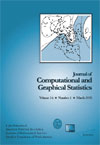Want to share your content on R-bloggers? click here if you have a blog, or here if you don't.In the continuation of my earlier post on computing evidence, I read a very interesting paper by Merlise Clyde, Joyee Ghosh and Michael Littman, to appear in JCGS. It is called  Bayesian adaptive sampling for variable selection and model averaging. The sound idea at the basis of the paper is that, when one is doing variable selection (i.e. exploring a finite if large state space) in setups where the individual probabilities of the models are known (up to a constant), it is not necessary to return to models that have been already visited. Hence the move to sample models without replacement called BAS (for Bayesian adaptive sampling) in the paper. The first part discusses the way to sample without replacement a state space whose elements and probabilities are defined by a binary tree, i.e.$f(mathbf{gamma})=prod_{j=1}^k rho_{j|

(The connection with variable selection is that each level of the tree corresponds to the binary choice between including and excluding one of the variables. The tree thus has 2k endpoints/leaves for k potential variables in the model.) The cost in updating the probabilities is actually in O(k) if k is the number of levels, instead of 2k because most of the branches of the tree are unaffected by setting one final branch to probability zero. The second part deals with the adaptive and approximative issues.

In a model selection setup, the posterior partial conditional probability of including variable i given the inclusion/exclusion of variables 1,…,i-1 is obviously unknown. The authors suggest to use instead an approximation based on the marginal posterior inclusion probabilities, namely for variable j$hatrho_j = sum_t f(mathbf{x}|mathfrak{M}_{mathbf{gamma}^t})gamma_j^t bigg/sum_t f(mathbf{x}|mathfrak{M}_{mathbf{gamma}^t})$

the frequency of the inclusion of variable j in the model along past iterations, with a possible shrinkage correction to avoid probability estimates equal to zero. (The motivation is the fundamental paper by Berger and Barbieri, 2004, Annals of Statistics, that shows the optimality of the median posterior probability model.) In this sense, the algorithm is adaptive. (In addition, there is a step to periodically calculate new marginal inclusion probabalities that replace the old sampling distribution at time t=0.) But it also is approximative in that the only convergence result is one by attrition, namely that the posterior partial conditional probabilities are exactly recovered after sampling all models. (The paper is associated with an R package called BAS.)